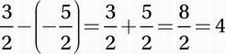# SAT Math Multiple Choice Question 641: Answer and Explanation

### Test Information

Question: 641

11. In the xy-plane, the graph of the line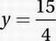intersects the graph of the equation y = x2 + x at two points. What is the distance between these two points?

• A.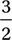• B.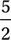• C.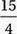• D. 4

Explanation:

D

Given equation:

y = x2 + x

Substitute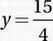: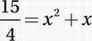Multiply by 4:

15 = 4x2 + 4x

Subtract 15:

0 = 4x2 + 4x - 15

Factor using the Product-Sum Method (Chapter 9, Lesson 4):

0 = (2x + 5)(2x - 3)

Use Zero Product Property (Chapter 9, Lesson 5):

2x + 5 = 0; 2x - 3 = 0

Solve each equation for x:

x = -5/2; x = 3/2

Therefore, the two points of intersection are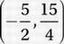and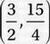, and the distance between these points is